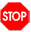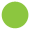06-parser-combinators

# Module 06: Parser combinators

• Write your team names here:

In practice, one rarely writes parsers from scratch like we have done on previous modules. Typically, one uses some sort of tool or framework for constructing parsers, which hides a lot of the complexity of dealing with lexing/tokenizing, precendence and associativity, etc., and allows you to focus more directly on the grammar you wish to parse.

In this module, we will explore a Haskell library for constructing parsers called `parsec`.

If you are using `repl.it` you should not need to do anything special to be able to use `parsec`; just make sure that you upload the provided file `Parsing.hs` to your project. If you have Haskell installed on your local machine, to install `parsec`, you should open a command prompt and type

``cabal install --lib parsec``

You should skim over the following code but you need not read it too closely at this point. Later parts of the module will direct you to read parts of it more carefully.

``````{-# LANGUAGE GADTSyntax #-}

-- Hide some standard operators so we can use
-- variants with more specific types (for now)
import Prelude hiding ((<\$>), (<\$), (<*>), (<*), (*>))

-- Parsing is a module I have provided for you which wraps up some
-- functionality of parsec into a somewhat easier/simpler interface.
import Parsing

-- Our old friend Arith
data Arith where
Lit :: Integer -> Arith
Add :: Arith -> Arith -> Arith
Sub :: Arith -> Arith -> Arith
Mul :: Arith -> Arith -> Arith
deriving (Show)

interpArith :: Arith -> Integer
interpArith (Lit i) = i
interpArith (Add e1 e2) = interpArith e1 + interpArith e2
interpArith (Sub e1 e2) = interpArith e1 - interpArith e2
interpArith (Mul e1 e2) = interpArith e1 * interpArith e2

lexer :: TokenParser u
lexer = makeTokenParser emptyDef

parens :: Parser a -> Parser a
parens     = getParens lexer

reservedOp :: String -> Parser ()
reservedOp = getReservedOp lexer

integer :: Parser Integer
integer    = getInteger lexer

whiteSpace :: Parser ()
whiteSpace = getWhiteSpace lexer

parseArithAtom :: Parser Arith
parseArithAtom = (Lit <\$> integer) <|> parens parseArith

parseArith :: Parser Arith
parseArith = buildExpressionParser table parseArithAtom
where
table = [ [ Infix (Mul <\$ reservedOp "*") AssocLeft ]
, [ Infix (Add <\$ reservedOp "+") AssocLeft
, Infix (Sub <\$ reservedOp "-") AssocLeft
]
]

arith :: Parser Arith
arith = whiteSpace *> parseArith <* eof

eval :: String -> Maybe Integer
eval s = case parse arith s of
Left _  -> Nothing
Right e -> Just (interpArith e)``````

## Token parsers

• Choose who will start out as the driver, and write their name here:

The first thing to consider is `lexer`, which is a `TokenParser`. For now, we are using a simple default `TokenParser`; later we will see how to customize it. Essentially, `lexer` is an automatically generated collection of special parsers which do the low-level work of tokenizing. The functions `getInteger`, `getParens`, etc. extract these individual parsers from `lexer`. We have extracted four such token parsers and given them names to make them easier to use: one to parse integers, one for whitespace, one for operators, and one to parse parenthesized things. (See the definition of `GenTokenParser` for a full list of the available token parsers.)

Parsers have a type like `Parser a`; for example, `integer` has type `Parser Integer`. This means it is a parser which consumes some part of a `String` and either returns a value of type `Integer` or fails with parse error. You can think of it like this:

`Parser a = String -> Maybe (a, String)`

although the actual definition is quite a bit more complicated. Note also that a `Parser a` is NOT actually a function.

You can use the `parseSome` function (provided in the `Parsing` module) to try a parser and see what part of the input it consumes and what part is left to be consumed by subsequent parsers. In other words, `parseSome` turns a `Parser` into a function like the one described above.

• Try the `integer` parser using `parseSome`. For example, you could evaluate `parseSome integer "23"` in GHCi. Explain how `integer` behaves. Be sure to try lots of different inputs: try spaces before and/or after an integer, try a negative sign, try non-digit characters before and after, etc. (You do not have to record the results of all your experiments.) Explain in detail what the `integer` parser does.

• Try each of the following and explain what they do. Just as with `integer`, be sure to try many different inputs.

• `reservedOp`

• `whiteSpace`

• `parens`

• In general, how do token parsers like `integer`, `reservedOp`, and `parens` handle spaces?## Parser combinators

• ROTATE ROLES and write the name of the new driver here:

The token parsers provide the primitive building blocks out of which we can construct more complicated parsers. Now we will explore some of the functions for building up more complex parsers. Such functions that allow building more complex things out of simpler parts are known as combinators.

• Try `integer <|> parens integer`. (As before, “try” means "use the `parseSome` function to try it on various example inputs.)

• What does `integer <|> parens integer` do?

• What is the type of `(<|>)`?

• What does `(<|>)` do in general?

• Now consider `Lit <\$> integer`.

• What does `Lit <\$> integer` do?

• What is the type of `(<\$>)`? Explain what this type means in your own words.

• What is the type of `Lit`?

• What is the type of `Lit <\$> integer`? What does it do?

• Try `integer *> integer`.

• What does `integer *> integer` do?

• What is the type of `(*>)`? Explain what this type means in your own words.

• What does `(*>)` do?

• What do you think `(<*)` does? Guess before trying it.

• Now try it. Were you right?

• Try `"I" <\$ integer`.

• What does `"I" <\$ integer` do?

• What is the type of `(<\$)`?

• What does `(<\$)` do?

• How is `(<\$)` similar to `(<*)`? How is it different?## Combinator exercises

• ROTATE ROLES and write the name of the new driver here:

• Write a parser which parses two integers, discards the first one, and returns the second wrapped in a `Lit` constructor. Be sure to test it!

• Write a parser which parses an integer surrounded by `~` on either side (for example, `"~23~"`) and returns the integer.

• Skim through the `parsec` documentation for the following modules:

Note, wherever you see something like `ParsecT s u m a` you should think of it as `Parser a` (`ParsecT s u m a` is a more general version of `Parser a`).

Pick one of the combinators you find and demonstrate its use. You will have to add an `import` statement to the top of this file of the form: `import Text.Parsec.SomeModule (combinatorYouChose)`## Building an Arith parser

• ROTATE ROLES and write the name of the new driver here:

Now we will explore how the token parsers and combinators are used to build a parser for the Arith language.

Note that we have defined two parsers of type `Parser Arith`, namely, `parseArith` and `parseArithAtom` (not counting `arith`, which we will discuss later). This is a common pattern when building parsers for languages with infix operators. The idea is that an “atomic” thing is something which forms an indivisible unit, which we know how to parse just by looking at the first token. A non-atomic thing might be more complicated; in particular, it might involve infix operators.

• Explain what `parseArithAtom` does.

Now look at the definition of `parseArith`. It uses a function provided by `parsec` called `buildExpressionParser`, which deals with parsing infix operators with various precedence levels and associativities (using similar algorithms to those explored in module 6).

• Explain what the parser `(Mul <\$ reservedOp "*")` does. What is its type?

Notice that `table` consists of a list with two elements, each of which is itself a list. The first list has one element which refers to `Mul`; the second list has two elements referencing `Add` and `Sub`.

• What do you think this list signifies?

• Test your theory by switching the order of the two lists, and doing some test parses. Were you right? (Then switch them back.)

Finally, take a look at the `arith` parser.

• What does the `eof` parser do? (Try it on some examples, and/or read its documentation.)

• What does `arith` do?

We have now explored the entire Arith parser. Let’s modify it a bit.

• Extend Arith with an exponentiation operator. Remember that exponentiation has higher precedence than multiplication and is right-associative. (The exponentiation operator in Haskell is `(^)`.) You will have to modify the `Arith` data type, the interpreter, and the parser. Be sure to test that it works correctly.

• Now extend Arith with prefix negation. For example, `"-(2+3)"` should evaluate to `-5`. Again, you should modify the `Arith` definition, parser, and interpreter. When modifying the parser, you can use `Prefix` instead of `Infix`. See the documentation for `Text.Parsec.Expr`.## The `(<*>)` operator

There is one more parser operator we need to learn about, namely, `(<*>)`. It wasn’t needed in the Arith parser but will often be useful.

• What is the type of `(<*>)`?

Unfortunately, the type of `(<*>)` does not give you a good sense of how to use it unless you have already spent a good deal of time thinking about higher-order functions, currying, and the like in functional programming. Instead, we’ll look at some examples.

``````add :: Integer -> Integer -> Integer
add x y = x + y``````
• Test the parser `(add <\$> integer <*> integer)`. What does it do?

(In fact, we could also have written `((+) <\$> integer <*> integer)`, which would do exactly the same thing.)

• Write a function `add3` which takes three `Integer`s and adds them.

• Now test the parser `(add3 <\$> integer <*> integer <*> integer)`. What does it do?

• In general, what do you think `(func <\$> parser1 <*> parser2 <*> ... <*> parserN)` does?

• Write a parser `adder :: Parser Arith` which expects to read two integer values, and returns an `Arith` value consisting of an `Add` node containing the two integers. For example:

``````parseSome adder "32 9 xyz"
Right (Add (Lit 32) (Lit 9), "xyz")``````## BIN parser

• ROTATE ROLES and write the name of the new driver here:

• Recall the BIN language from module 4. As a reminder, the concrete syntax of BIN looks like this:

``````  <bin> ::= '#'
| '(' <bin> <bin> ')'``````

Write a parser for BIN using parsec. A few hints:

• Define `symbol = getSymbol lexer` and use it to parse the `#` character. Using `reservedOp` will not work since it wants to treat `##` as a single operator.

## Feedback

• How long would you estimate that you spent working on this module?

• Were any parts particularly confusing or difficult?

• Were any parts particularly fun or interesting?

• Record here any other questions, comments, or suggestions for improvement.